Courses

# Introduction: The Moment Distribution Method - 2 Civil Engineering (CE) Notes | EduRev

## Civil Engineering (CE) : Introduction: The Moment Distribution Method - 2 Civil Engineering (CE) Notes | EduRev

The document Introduction: The Moment Distribution Method - 2 Civil Engineering (CE) Notes | EduRev is a part of the Civil Engineering (CE) Course Structural Analysis.
All you need of Civil Engineering (CE) at this link: Civil Engineering (CE)

Example 18.1
A continuous prismatic beam ABC (see Fig.18.2a) of constant moment of inertia is carrying a uniformly distributed load of 2 kN/m in addition to a concentrated load of 10 kN. Draw bending moment diagram. Assume that supports are unyielding.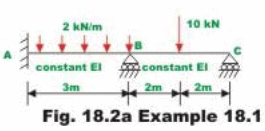Solution
Assuming that supports B and C are locked, calculate fixed end moments developed in the beam due to externally applied load. Note that counterclockwise moments are taken as positive.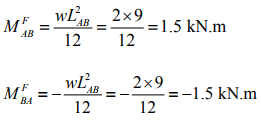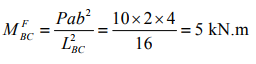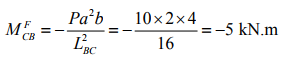(1)

Before we start analyzing the beam by moment-distribution method, it is required to calculate stiffness and distribution factors.

KBA = 4EI/3

KBC = 4EI/4

At B: ∑ K = 2.333 EI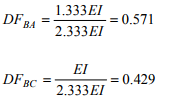At C: ∑ K = EI

DFCB = 1.0

Note that distribution factor is dimensionless. The sum of distribution factor at a joint, except when it is fixed is always equal to one. The distribution moments are developed only when the joints rotate under the action of unbalanced moment. In the case of fixed joint, it does not rotate and hence no distribution moments are developed and consequently distribution factor is equal to zero. In Fig.18.2b the fixed end moments and distribution factors are shown on a working diagram. In this diagram B and C are assumed to be locked.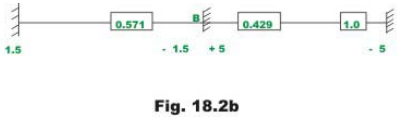Now unlock the joint C. Note that joint C starts rotating under the unbalanced moment of 5 kN.m (counterclockwise) till a moment of -5 kN.m is developed (clockwise) at the joint. This in turn develops a beam end moment of +5 kN.m (MCB). This is the distributed moment and thus restores equilibrium. Now joint C is relocked and a line is drawn below +5 kN.m to indicate equilibrium. When joint C rotates, a carry over moment of +2.5 kN.m is developed at the B end of member BC.These are shown in Fig.18.2c.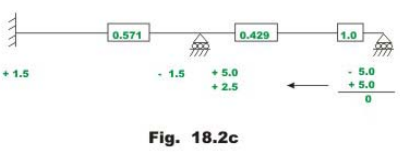When joint B is unlocked, it will rotate under an unbalanced moment equal to algebraic sum of the fixed end moments(+5.0 and -1.5 kN.m) and a carry over moment of +2.5 kN.m till distributed moments are developed to restore equilibrium. The unbalanced moment is 6 kN.m. Now the distributed moments MBC and MBA are obtained by multiplying the unbalanced moment with the corresponding distribution factors and reversing the sign. Thus, MBC = -2.574 kN.m and MBA = -3.426 kN.m . These distributed moments restore the equilibrium of joint B. Lock the joint B. This is shown in Fig.18.2d along with the carry over moments.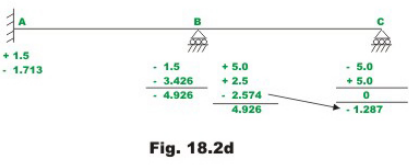Now, it is seen that joint B is balanced. However joint C is not balanced due to the carry over moment -1.287 kN.m that is developed when the joint B is allowed to rotate. The whole procedure of locking and unlocking the joints C and B successively has to be continued till both joints B and C are balanced simultaneously. The complete procedure is shown in Fig.18.2e.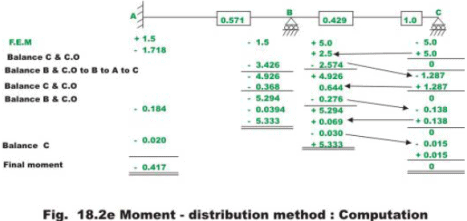The iteration procedure is terminated when the change in beam end moments is less than say 1%. In the above problem the convergence may be improved if we leave the hinged end C unlocked after the first cycle. This will be discussed in the next section. In such a case the stiffness of beam BC gets modified. The above calculations can also be done conveniently in a tabular form as shown in Table 18.1. However the above working method is preferred in this course.

Table 18.1 Moment-distribution for continuous beam ABC

 Joint A B C Member AB BA BC CB Stiffness 1.333EI 1.333EI EI EI Distribution factor 0.571 0.429 1.0 FEM in kN.m +1.5 -1.5 +5.0 -5.0 Balance joints C ,B and C.O -1.713 -3.426 +2.5 -2.579 +5.0 0 -4.926 +4.926 -1.287 Balance C and C.O. +0.644 1.287 Balance B and C.O. -0.368 -0.276 -0.138 Balance C -5.294 +5.294 0.138 C.O. +0.069 0 Balance B and C.O -0.039 -0.030 -0.015 Balance C +0.015 Balanced moments in kN.m -5.333 +5.333 0

Modified stiffness factor when the far end is hinged

As mentioned in the previous example, alternate unlocking and locking at the hinged joint slows down the convergence of moment-distribution method. At the hinged end the moment is zero and hence we could allow the hinged joint C in the previous example to rotate freely after unlocking it first time. This necessitates certain changes in the stiffness parameters. Now consider beam ABC as shown in Fig.18.2a. Now if joint C is left unlocked then the stiffness of member BC changes. When joint B is unlocked, it will rotate by θB1 under the action of unbalanced moment MB . The support C will also rotate by θC1 as it is free to rotate. However, moment MCB = 0. Thus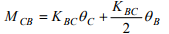(18.7)

But, MCB = 0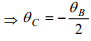(18.8)

Now,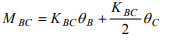(18.9)

Substituting the value of θC in eqn. (18.9),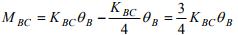(18.10)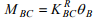(18.11)

The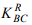is known as the reduced stiffness factor and is equal tois known as the reduced stiffness factor and is equal to 3/4 KBC . Accordingly distribution factors also get modified. It must be noted that there is no carry over to joint C as it was left unlocked.

Example 18.2
Solve the previous example by making the necessary modification for hinged end C.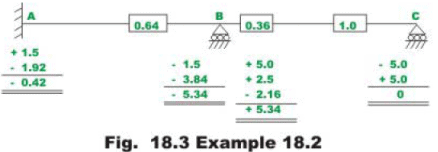Fixed end moments are the same. Now calculate stiffness and distribution factors.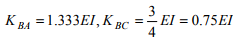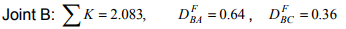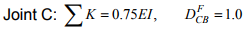All the calculations are shown in Fig.18.3a

Please note that the same results as obtained in the previous example are obtained here in only one cycle. All joints are in equilibrium when they are unlocked. Hence we could stop moment-distribution iteration, as there is no unbalanced moment anywhere.

Example 18.3
Draw the bending moment diagram for the continuous beam ABCD loaded as shown in Fig.18.4a.The relative moment of inertia of each span of the beam is also shown in the figure.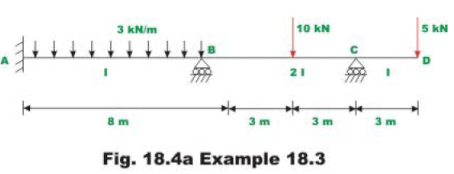Solution
Note that joint C is hinged and hence stiffness factor BC gets modified. Assuming that the supports are locked, calculate fixed end moments. They are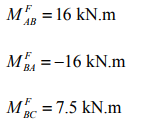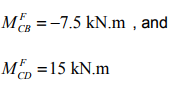In the next step calculate stiffness and distribution factors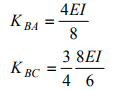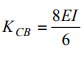At joint B:

∑ K = 0.5EI + 1.0EI = 1.5EI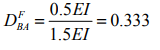At C: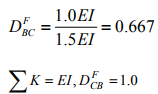Now all the calculations are shown in Fig.18.4b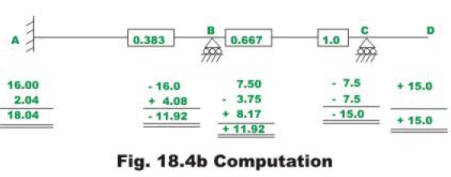This problem has also been solved by slope-deflection method (see example 14.2).The bending moment diagram is shown in Fig.18.4c.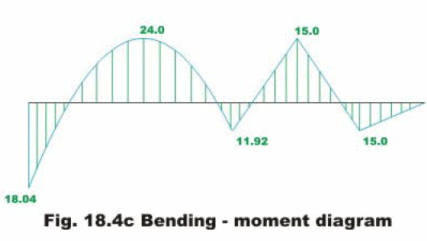Summary

An introduction to the moment-distribution method is given here. The momentdistribution method actually solves these equations by the method of successive approximations. Various terms such as stiffness factor, distribution factor, unbalanced moment, distributing moment and carry-over-moment are defined in this lesson. Few problems are solved to illustrate the moment-distribution method as applied to continuous beams with unyielding supports.

Offer running on EduRev: Apply code STAYHOME200 to get INR 200 off on our premium plan EduRev Infinity!

## Structural Analysis

30 videos|122 docs|28 tests

,

,

,

,

,

,

,

,

,

,

,

,

,

,

,

,

,

,

,

,

,

;